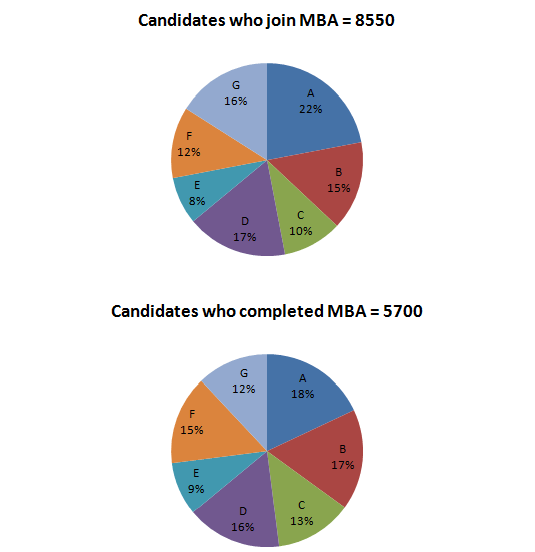Direction for the Question: Study the following pie-charts to answer these question.

Distribution of Candidates who joined M.B.A. Programme and successfully completed for institutes A to G.Question 1: Which institute has the highest percentage of candidates who completed the M.B.A programme out
of the candidates that joined?

(a) C
(b) B
(c) F
(d) E

Question 2: What percentage of candidates completed the M.B.A. programme from Institute E out of the total number of candidates that joined the same institute?
(a) 62.5%
(b) 60%
(c) 75%
(d) 50%

Question 3: What is the ratio of candidates who successfully completed the M.B.A. programme to the candidates who joined from Institute G?
(a) 3 : 4
(b) 1 : 2
(c) 6 : 11
(d) 9 : 17

Question 4: What percentage of candidates completed the M.B.A. programme out of the candidates who joined for Institutes Band C together?
(a) 64%
(b) 75%
(c) 90%
(d) 80%

Question 5: The number of candidates who completed the M.B.A. programme from A and D Institutes together exceeds the number of candidates who joined Institutes C and E together by
(a) 304
(b) 278
(c) 399
(d) 369

Since the ratio of total candidates who joined MBA to the total students

who completed will be constant throughout, only the percentage value ratios need to be considered.

For C we have the ratio 13/10 = 1.3

For B we have the ratio 17/15 = 1.13

For F we have the ratio 15/12 = 1.25

For E we have the ratio 9/8 = 1.125

As the ratio is maximum for C, so C has the highest  percentage of candidates who completed the M.B.A program out of the candidates that joined.

The number of candidates who joined the institute E = 8/100 x 8550 = 684

The number of candidates who completed the course = (9/100) x 5700 – 513

The required percentage = (513/684) x 100 = 75%

The number of candidates who joined the institute G = 16% of 8550 = 1368

The number of candidates who completed the course = 12% of 5700 = 684

So, the required ratio is 684: 1368 or 1: 2

The correct option is (b)

The total number of candidates who joined the institute B and C together = 25% of 8550 = 2137.5

The total number of candidates who completed the programme = 30% of 5700 = 1710

Hence, the required percentage = (1710/2137.5) x 100 = 80%

The correct option is (d)

Answer 5: (c) The number of candidates who completed the M.B.A. programme from A and D =34% of 5700 = 1938.

The number of candidates who joined Institutes C and E together=18% of 8550 = 1539

The required difference = 1938 – 1539 = 399

The correct option is (c)

### Want to explore more Data Interpretation Sets?

Get Posts Like This Sent to your Email
Updates for Free Live sessions and offers are sent on mail. Don't worry: we do not send too many emails..:)
Get Posts Like This Sent to your Email
Updates for Free Live sessions and offers are sent on mail. Don't worry: we do not send too many emails..:)